# msamp: Sample size for detecting microbial contamination

## Introduction

The msamp package estimates the sample size needed to detect microbial contamination in a lot with a user-specified probability of detection and user-specified analytical sensitivity. Distributions of microbial contamination in the lot addressed in this package are:

• homogeneous: assumes bacteria are randomly and uniformly distributed (Poisson)
• heterogeneous: assumes bacteria are unevenly distributed (Poisson-Gamma)
• localized: bacteria are present in clusters (Zero-inflated Poisson).

## p()

calculates the probability that a single sample unit is contaminated above the user-specified microbial target level.

## n()

calculates the number of samples (n) needed to detect microbial contamination, above the user-specified target level, in the entire lot and the total cost associated with this number of samples (cost_tot).

## plotn()

plots the desired probability of detecting microbial contamination in the lot above the target level , prob_det, (y-axis) vs. the estimated sample size n (x-axis).

## Function Arguments

The functions use the following arguments:

• C (CFU/g): the suspected concentration of bacteria in the lot
• w (g): the weight of the sample unit.Grams (g) are used uniformly in this work.
• G (CFU/g): the target value of bacterial concentration to detect – may be regulatory limit or a risk-based value. For example if one wants to detect microbial contamination in the lot above 5 CFU/g, then G=5.
• Sens: the sensitivity of the analytical or laboratory test used to detect bacteria, e.g. if the sensitivity of the analytic test is 90%, Sens= 0.9. 0 $$\le$$ Sens $$\le$$ 1
• D: the type of bacterial distribution in the lot. One of “homogeneous”, “heterogeneous” or “localized”
• prob_det: the desired probability of detecting contamination in the lot - - set to 0.9 by default. For n() function only.
• samp_dollar (dollars): the cost per sample unit. For n() function only.
• lot_dollar (dollars): the fixed sampling cost per lot. For n() function only.

In this package, the weight of the sample unit, w, corresponds to the weight of the analytical unit; i.e. the amount of product that is tested. The distribution in a sample unit is assumed to be the same as the distribution in the product lot. For example, if the bacteria is localized in the lot, it is assumed that the bacteria is also localized in the sample unit. Although this assumption may be challenging to achieve, to that end samples should be selected such that they are representative of the product lot, akin to a random sampling scheme.

## Function Details

p()

The probability of a single sample unit being contaminated above the target microbial level, G, is calculated using the Poisson distribution for a homogeneous distribution of bacteria, the negative binomial (NB) (i.e. Poisson gamma) distribution for a heterogeneous distribution of bacteria, and the zero-inflated Poisson distribution for a localized or clustered distribution of bacteria. This probability of contamination for a single sample unit is then multiplied by the sensitivity of the analytical test, Sens.

p(C,w,G,Sens,D,r=NULL,f=NULL) where C, w, G, r, f, Sens and D are defined in Function Arguments and

• mu ($$\mu)$$ is the suspected mean level of contamination (# CFU’s) for a single sample unit: $$\mu$$ = C * w where C and w are defined above.
• g is the target level of contamination (# CFU’s) to detect in a single sample unit: g = round(G * w,0) where G and w are defined above
• r is the postulated level of heterogeneity or dispersion for the heterogeneous case: higher values mean greater heterogeneity. r $$\gt$$ 0.
• f is the postulated fraction of the lot which is contaminated for the localized case. 0 $$\le$$ f $$\le$$ 1.

The probability of a single sample unit being contaminated above the target level with sensitivity Sens is:

For the homogeneous case:
p = Sens $$\times$$ ppois(g, mu, lower.tail = FALSE, log.p = FALSE) where ppois is $P(X \gt g) = \sum_{x \gt g} \frac{e^{-\mu} \mu^x}{x!}$

For the heterogeneous case:
p = Sens $$\times$$ pnbinom(g, mu=mu, size=r,lower.tail = FALSE, log.p = FALSE). The Poisson-gamma distribution is the pertinent distribution, and is equivalent to the NB distribution (pnbinom). $P(X \gt g) = \sum_{x \gt g} \frac{\Gamma(r+x)}{x!\Gamma(r)}(\frac{r}{r+\mu})^{r} (\frac{\mu}{r+\mu}){^x}$ This is the explicit parameterization of the NB distribution in terms of the dispersion parameter (r, defined above), where $variance= \mu+\mu^2/r$

For the localized case:

p = Sens $$\times$$ f $$\times$$ ppois(g, mu, lower.tail = FALSE, log.p = FALSE) where ppois is the Poisson distribution functon (defined above) and f is defined above as the fraction of the lot which is contaminated $f* P(X \gt g) = \sum_{x \gt g} \frac{e^{-\mu}\mu^x}{x!}$ and $f=1-P(X=0)$

## Example using p() function

library(msamp)

#Example: Sample of 25 grams (w=25) is collected and analyzed using an analytical test with sensitivity of 90% (Sens=.9), to detect at least 5 CFU's/g (G=5). The suspected or postulated level of contamination in the lot is 4 CFU's/g (C=4)
#homogeneous case
p(C=4,w=25,G=5,Sens=.9,D="homogeneous",r=NULL,f=NULL)
# 0.006117884
#heterogeneous case-- dispersion, r, is postulated as 2
p(C=4,w=25,G=5,Sens=.9,D="heterogeneous",r=2,f=NULL)
# 0.2576463
#localized case -- 30% of the lot is postulated to be contaminated
p(C=4,w=25,G=5,Sens=.9,D="localized",r=NULL,f=.3)
# 0.001835365
#heterogeneous case-- dispersion, r, is postulated as 100
p(C=4,w=25,G=5,Sens=.9,D="heterogeneous",r=200,f=NULL)
# 0.02037385, closer to p for homogeneous case. As r increases, NB converges to Poisson 

The Poisson and NB distributions are both skewed right, however the NB is more skewed to the right than the Poisson (Simon 1963). For lot levels with contamination (C) less than the target level (G), the probability of a single sample unit being contaminated above G is greater for the heterogeneous (NB) case than for the case homogeneous (Poisson). For lot levels with contamination (C) greater than G, the probability of a single sample unit being contaminated above G is greater for the homogeneous case than the heterogeneous case.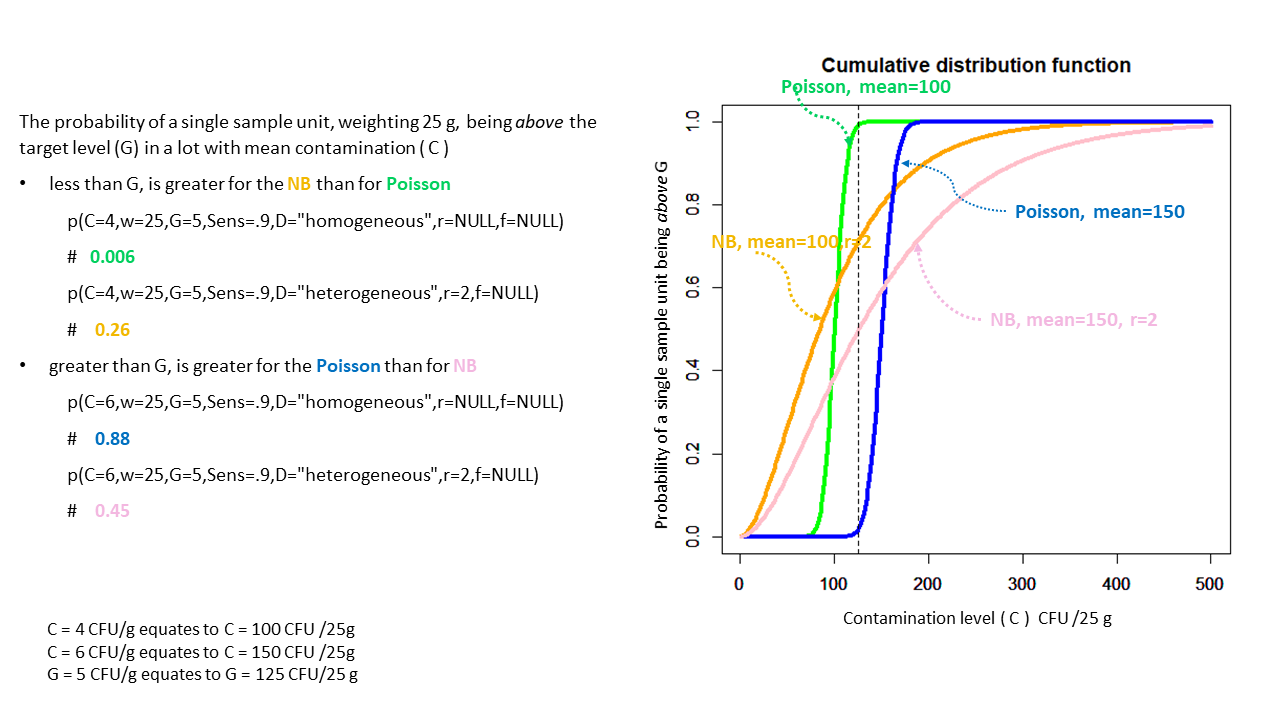As the dispersion of the NB increases, the NB converges to the Poisson.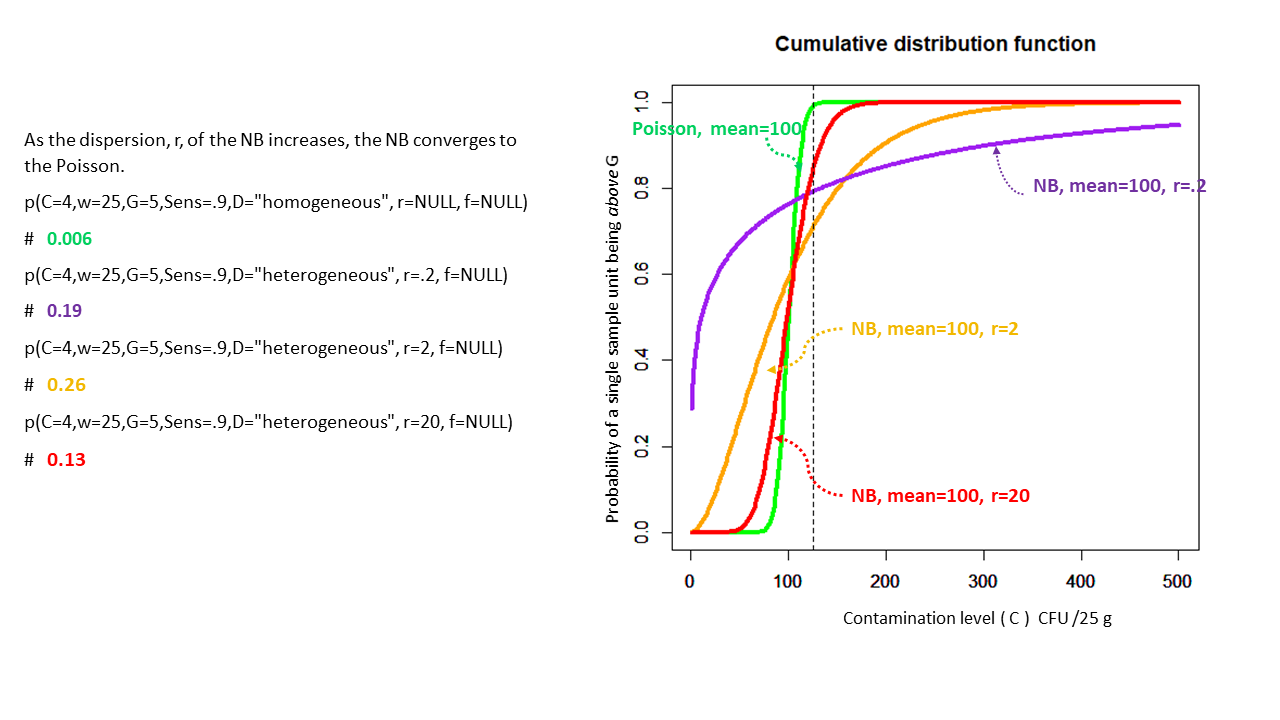## n()

The number of samples (n) needed to detect microbial contamination within a lot above the user-specified target level, where the probability, p, of a single sample unit being contaminated above target level is calculated by the function p()

n(C,w,G,Sens,D,r=NULL,f=NULL,prob_det=0.9,samp_dollar,lot_dollar) where C, w, G, r, f, Sens and D are defined above and

• prob_det is the probability of detecting contamination above target level in the lot, i.e. the probability of sampling at least one unit contaminated above the target level. prob_det is set to 0.9 by default.
• samp_dollar is the cost per sample unit in $• lot_dollar is the fixed sampling cost per product lot in$

The sample size is derived from the binomial distribution for selecting at least one sample unit whose probability of being contaminated above the target level is p: $P(X \gt 0) = \sum_{x \gt 0} \binom{n}{x} p^x (1-p)^{n-x} = 1- P(X = 0) = 1- (1-p)^{n}$ Specifying a desired probability of detecting contamination in the lot above the target level, i.e. selecting at least one sample unit contaminated above the target level: $prob\_det= 1- (1-p)^{n}$ and solving for n (rounded up), the formula for the requisite sample size is: $n= ceiling(log(1 - prob\_det) / log(1 - p))$ The total cost associated with taking n samples is: $lot\_dollar + n * samp\_dollar$

## Example continued, using n() function

Example continued:desired probability of picking at least one sample unit contaminated above the target level is 0.9 (prob_det=0.9), the cost of a single sampling unit is $100 (samp_dollar=100), the fixed cost for sampling the entire lot is$200 (lot_dollar=200).

#homogeneous case
n(C=4,w=25,G=5,Sens=.9,D="homogeneous",r=NULL,f=NULL,prob_det=0.9,samp_dollar=100,lot_dollar=200)
# n=376, total cost=$37,722 #heterogeneous case n(C=4,w=25,G=5,Sens=.9,D="heterogeneous",r=10,f=NULL,prob_det=0.9,samp_dollar=100,lot_dollar=200) # n=12, total cost=$1,319
#localized case
n(C=4,w=25,G=5,Sens=.9,D="localized",r=NULL,f=.3,prob_det=0.9,samp_dollar=100,lot_dollar=200)
# n=1,254 , total cost=$125,541 Decreasing the sample unit weight, w, results in lowering the target level to detect in that sample unit , thereby increasing the probability that any given sample unit would be above the target level and relaxing the sample size requirement. #homogeneous case with w=10 n(C=4,w=10,G=5,Sens=.9,D="homogeneous",r=NULL,f=NULL,prob_det=0.9,samp_dollar=100,lot_dollar=200) # n=48, total cost=$4,945. n is less than that required when w=25.

## plotn()

Explores the relationship between prob_det and sample size n by plotting prob_det (y-axis) vs. n (x-axis) where details for n are found under the Details section for function n() and whose value is calculated for each prob_det.

plotn(C,w,G,Sens,D,r,f) where C, w, G, r, f, Sens, D and prob_det are defined above.

## Example continued, using plotn() function

Example continued:Plot probability of detection (prob_det) vs. sample size (n)
#homogeneous case
plotn(C=4,w=25,G=5,Sens=.9,D="homogeneous",r=NULL,f=NULL)
# n=376 for probability of detection=0.9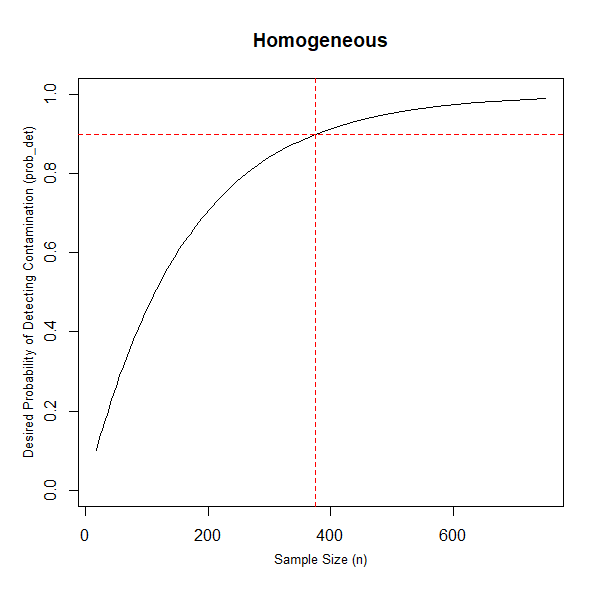#heterogeneous case
plotn(C=4,w=25,G=5,Sens=.9,D="heterogeneous",r=10,f=NULL)
# n=12 for probability of detection=0.9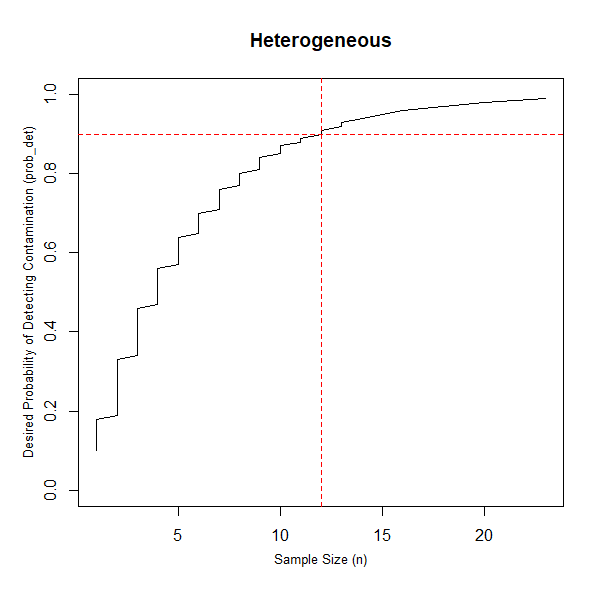#localized case
plotn(C=4,w=25,G=5,Sens=.9,D="localized",r=NULL,f=.3)
# n=1,254 for probability of detection=0.9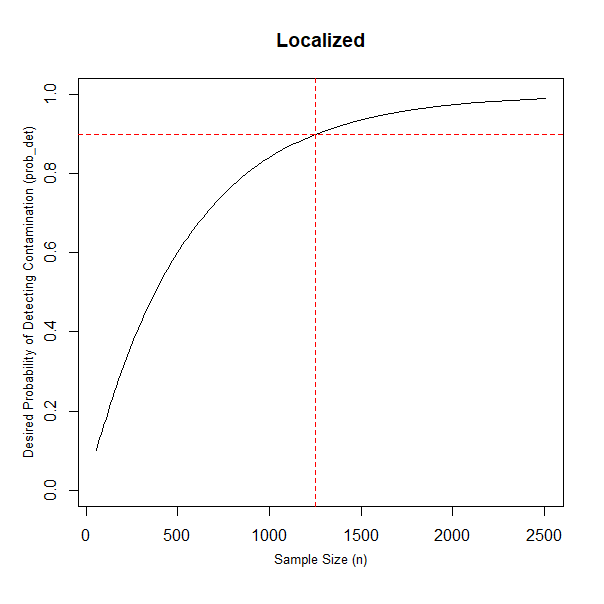## Conclusion

Bacterial contamination in food is not always evenly distributed in product lots but sample size estimations often assume that it is. To better support the sampling of food product lots, the msamp package was developed to account for additional bacterial distributions, such as heterogeneous or localized. This package can be used to estimate sample sizes for different scenarious such as the type of bacterial distribution (homoegeneous, heterogeneous or localized) and varying levels of bacterial contamination.